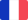•# Fifth Conference on Optimization Techniques. Rome 1973

Autor: Roberto Conti

Identification of systems subject to random state disturbance.- Adaptive compartimental structures in biology and society.- On the optimal size of system model.- Information-theoretic methods for modelling and analysing large systems.- A new criterion for... Viac o knihe

Na objednávku, dodanie 2-4 týždne

28.04 €

bežná cena: 32.99 €

## O knihe

Identification of systems subject to random state disturbance.- Adaptive compartimental structures in biology and society.- On the optimal size of system model.- Information-theoretic methods for modelling and analysing large systems.- A new criterion for modeling systems.- Stochastic extension and functional restrictions of ill-posed estimation problems.- Regression operator in infinite dimensional vector spaces and its application to some identification problems.- An approach to identification and optimization in quality control.- Identification de Domaines.- The modelling of edible oil fat mixtures.- Free boundary problems and impulse control.- A convex programming method in Hilbert space and its applications to optimal control of system described by parabolic equations.- About some free boundary problems connected with hydraulics.- Methode de Decomposition Appliquee au Controle Optimal de Systemes Distribues.- Approximation of optimal control problems of systems described by boundary-value mixed problems of Dirichlet-Neumann type.- Control of parabolic systems with boundary conditions involving time-delays.- Characterization of cones of functions isomorphic to cones of convex functions.- Necessary conditions and sufficient conditions for pareto optimality in a multicriterion perturbed system.- A unified theory of deterministic two-players zero-sum differential games.- About optimality of time of pursuit.- Algebraic automata and optimal solutions in pattern recognition.- A new feature selection procedure for pattern recognition based on supervised learning.- A classification problem in medical radioscintigraphy.- The dynamic clusters method and optimization in non hierarchical-clustering.- A maximum principle for general constrained optimal control problems - an Epsilon Technique approach.- Optimal control of systems governed by variational inequalities.- On determining the submanifolds of state space where the optimal value surface has an infinite derivative.- Control of affine systems with memory.- Computational methods in Hilbert space for optimal control problems with delays.- Sufficient conditions of optimality for contingent equations.- Variational approximations of some optimal control problems.- Norm perturbation of supremum problems.- On two conjectures about the closed-loop time-optimal control.- Coupling of state variables in the optimal low thrust orbital transfer problem.- Optimization of the ammonia oxidation process used in the manufacture of nitric acid.- Stochastic control with at most denumerable number of corrections.- Design of optimal incomplete state feedback controllers for large linear constant systems.- Control of a non linear stochastic boundary value problem.- An algorithm to estimate sub-optimal present values for unichain markov processes with alternative reward structures.- Some recent developments in nonlinear programming.- Penalty methods and augmented Lagrangians in nonlinear programming.- On inf-compact mathematical programs.- Nonconvex quadratic programs, linear complementarity problems, and integer linear programs.- A widely convergent minimization algorithm with quadratic termination property.- A heuristic approach to combinatorial optimization problems.- A new solution for the general set covering problem.- A theoretical prediction of the input-output table.- An improved algorithm for pseudo-boolean programming.- Numerical algorithms for global extremum search.- Gradient techniques for computation of stationary points.- Parameterization and graphic aid in gradient methods.- Les Algorithmes de Coordination dans la Methode Mixte d'Optimisation a Deux Niveaux.- Applications of decomposition and multi-level techniques to the optimization of distributed parameter systems.- Attempt to solve a combinatorial problem in the continuum by a method of extension-reduction.

• Vydavateľstvo: Springer Berlin Heidelberg
• Rok vydania: 1973
• Formát: Paperback
• Rozmer: 235 x 155 mm
• Jazyk: Francúzsky jazyk
• ISBN: 9783540065838

Generuje redakčný systém BUXUS CMS spoločnosti ui42.